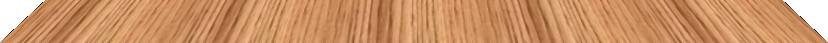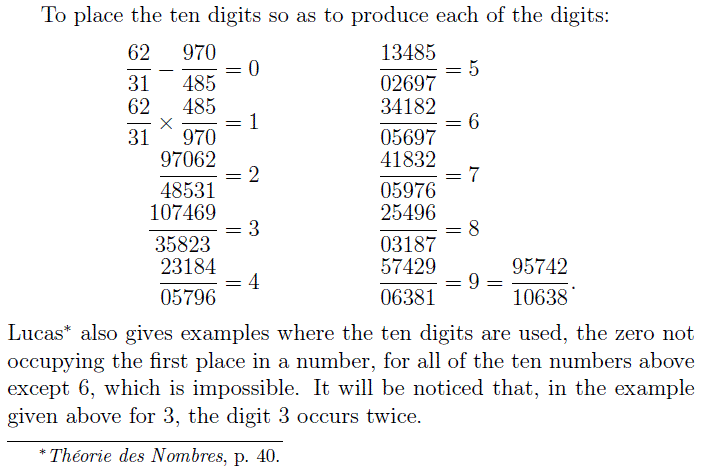#### Can you construct an expression for 100 which uses all the digits 1 to 9?

1

2

3

4

5

6

7

8

9###### That's correct. Can you find any other ways of doing it?
• You can put numbers together to make larger numbers, for example. 53, 127 etc.
• Don't forget the order of operations, BIDMAS, when evaluating your expressions.
• Surprisingly there's a solution in which all of the digits remain in numerical order.

## A Mathematics Lesson Starter Of The Day

• Jake, Patana
•
• I have found 1+2+3+4+5+6+7+8x9 = 100
• Lewis Crosby, Doncaster
•
• I have found one too 198-73-26+5-4=100
•
• Another solution for this is 56+49-2-3+8-7-1=100
•
• I have got found another solution for this starter =
6x7x2+8-3+1+9-4+5
• Holly, Whitby High, Ellesmere Port
•
• Another Solution: (5x6)+(4x9)+(8x3)+7+2+1 = 100
• Reece & Ashlee, Ellesmere Port
•
• Two more solutions without brackets from Whitby High:

123-4-5-6-7+8-9 = 100

69+31+8+5-7-4-2 = 100
• James Wellington-Smith, Lea Moor High
•
• 231-(9x8)-(6x5)-7-4
• Mr Heeley's Krew, Rawthorpe in da Hudd of field
•
• We found (1+9+2+8) x 5 + (3+7) - (4+6).

We think that this is the best answer because simplicity is the key!

God bless you - Maths rocks
• Year 6, BIS Jakarta
•
• We found many solutions including
9 * 4 * 2 + 8 + 7 + 3 + 5 + 6 - 1 = 100
9 * 8 + 7 + 6 + 5 + 4 + 3 + 2 + 1 = 100
9 * 8 + 4 * 7 - 5 * 2 + 6 + 3 + 1 = 100
• Year 11- Mr Kelly's class, Cross and Passion College
•
• We found another solution for ALL FOR 100

98+7-6+5-4+3-2-1=100
• Giovanni Fernandez, The British School of Quito, Ecuador
•
• 2 x (7 x 8 x 9 - 456) + 3 + 1
• Tim Channer, The British School of Quito, Ecuador
•
• (9 x 8) + (7 x 6) - (5 x 4) + (3 x 2 x 1)
• Montae, Mrs. Shelburne's Period 3/6 class, Hayward Middle School, Springfield, Ohio
•
• Here is Montae's solution...he found it in less than 5 minutes and creatively used exponents!

5^2 x 4 - 9 + 6 + 3 - 7 + 8 - 1
• Ashleigh Reid, S2 Lochend Community High School
•
• I found this way of getting the answer:

92 + 6 + 3 - 4 + 5 - 1 + 7 - 8
• Ciaran Donegan, Harrytown High School, Stockport, 8H
•
• I found (51+4+3) x 2 -9-8 + 7 -6
• Mrs Simpson, Torry Academy, Aberdeen
•
• 2D1 Maths Class at Torry Acadmey found this way:

89+7+6-5+(2*3)-4+1
• Andy Rowe, Knowles Hill School Devon
•
• Cameron Houdmont in Year 7 got this one

(7 + 3) x 9 + 5 + 4 + 1 + 8 - 6 - 2
• Ms Winters, Dubai English Speaking College
•
• Here are our answers from class 7DE...

9x8+1+2+3+4+5+6+7

6x3x5+12+4+(9-8)-7

9x8+7+1+5x6-(4x3-2)
• Miss Train, Saints Peter and Paul
•
• The first to get it in my class was Emma M in under 20 seconds - here's her solution:
[(1+2+3+4) x 9] + 5 + 6 + 7 - 8 = 100
• Jodie, Lauren, Hayley, Harrytown High School Stockport
•
• 123+45-67+8-9 Jodie Reilly 9T
98-7-6+5+4+3+2+1 Lauren Hill 9W
(1+2+3+4+5)x6-7+8+9 Hayley Ryder 9H
• Jack Houghton, Sydney, Australia
•
• I got 123+45-67+8-9
• Martin Vaughn, Central Sussex college haywards heath
•
• 8x9 +7 +1 +5 +6 +4 +3 +2 =100
•
12+34+59-6+8-7
• Miss Mian, Bishop Ramsey School, Ruislip
•
• Daniela got the total by doing
68 + 23 + 15 -4 +7 - 9

and Amy got it by doing

(9x8) + 1 + 2 + 3 + 4 + 5 + 6 + 7
• Josh Atkin, Dunbar Grammar
•
(98-7) +1 -4 +6 -2 +3+5=100
•

• Mark Daniels, Wissahickon Middle School
•
• Nicole from Mr. Daniels' Pre-Alg. Support class,WMS, Ambler,PA did this-
4(5+7+8) + 1(2+3+6+9)

Mr. Daniels offered this-
2^5 + (8x9-4) + 13-(6+7)
• Mrs Simpson and class 4M4, Torry Academy, Aberdeen
•
• Vincent found 963-852-7-4x1
Emma found 6x9x2-8+4+1-3+5-7
Shelby found 9x6+34+7+8-5+2x1
Jasmine found 9x7+(6x8)-5-4-3-1+2
• Liam Hutchison, Lochend Community High School in Glasgow
•
• I calculated it this way

(9x7) + 6 + 1 + (5 x 2) + (4 x 3) + 8
• Ellen Moore, KNGS, Birmingham,UK
•
• This works!
(9+1)-(2+8)+(3+7)+(4+6)*5=100
• Miss Samantha Rhodes, Filsham Valley school, Hastings
•
• In 1 class, I had 5 different correct solutions.
8x9+67+1-45+2+3 =100 (Charlotte Avery, year 8)
5x19+34-28-7+6=100 (Sam Davies, year 8)
3x4x6+2x9+(8-7+1)x5=100 (Sophie Fairall, year 8)
8x9+1+2+3+4+5+6+7=100 (Charlotte Rudd, year 8)
156-78+29-3-4=100 (James Bisson, year 8)
• Mr Jordan Fry, Laurence Jackson School, Guisbrough
•
• We got 5*9=45, 45+7=52, 52*2=104, 104-6=98, 98+4=102, 102-3=99, 99+1=100
•
• 78+24+9-6-5+3-2-1=100
• Soumya, Croydon
•
• 1+8x9+2+7+6+5+3-4=100.
• Form 7Y, Harrytown Catholic High School, Stockport
•
• Amy Booth found 9x8 + 7 + 6 + 5 + 4 + 3 + 2 + 1 = 100
James Wilson (9x8) + 7 + 6 + 5 + (4 x 3) - (2 X 1) = 100.
• Miss Marcham And 10f1, Prince Henry's High School, Evesham
•
• We got (6+4)x(8+9-7+5-3-2)x1 = 100.
• Mrs J A Thompson, Mexborough School Specialist Science College
•
• Two different answers were found in 10 minutes. 7P SPECTRUM group
The first was 1 + 2 + 3 + 4 + 5 +6 + 7 + (8x9) = 100 by Ashley Finney
and (1+7+2) x 9 + 8 + (4x5)- (3x6) = 100 by Emma Rodgers.
• Mitchel, Brocket
•
• Another way of doing it is
9-1=8, 8-2=6,7-3=4, 6-4=2, then
8+6+4+2=20x5=100.
• Y Ramsamy, Phoenix College, Reading, UK
•
• My pupils came up with the following solutions:
(124-3-5-6-8-9)+7 = 100 Ryan Walsh
(5x7x2x1)+3+4+6+8+9 = 100 Moin Khan
Y Ramsamy
• Jerry & Tom, Fukien School (HK)
•
• (1+2x3x4)x5-6*7+8+9=100.
• Thomas, Cabrach
•
• This wasn't easy but I found
9x8+7+(6x4)-5+1+3-2=100.
•
• Connor found 4x5x6-7-8-9-1+2+3
Brandon found 24x5-9-8-7+6+1-3
Brandon found 9x8+1+2+3+4+5+6+7.
• Mrs Barnett & 8x1, Bredon Hill Middle School, Evesham
•
• Our simplest answer was (9x8)+7+6+5+4+3+2+1 = 100.
• Greg Patko Yr8, Murray Park, Derby
•
• (9+8) x 7 - 6 x 5 + 4 x 3 + 1 - 2
(1 + 9 + 2 + 8 + 3 + 7 - 6 - 4) x 5.
• Mike Drewniak, Kewaskum Middle School
•
• Dinara found: 91+82-67-5-4+3.
• Beacon Community College, Crowborough, 8XMa3
•
• (6+4)x9+7+3+1+2+5-8=100.
• David Clowes, St Peter's Academy
•
• I got 7x5x4-9x3-8-6+2-1.
• Max, Hethersett Junior
•
• 12x5+39+6-8+7-4=100.
• 9 (the Better Half) 1, Chellaston Academy, Derbyshire
•
• Anneli and Molly: 9(6+3)+1+8+7+2+(5-4)
May: 2(6x9-3-1+8-5-7+4)
Rhys: 7x9+4x3+5+6x2+8x1
David: 1(932-845)+6+7
Eve: 5x6+87+1-9-4-3-2
Ashley: 9x8+7+6+5+4x3-2x1.
• 7ax1, Alexandra Park School, London
•
• Susie, Vinesha and Tommy : 8 x 9 + 7 + 1 + 6 + 5 + 4 + 2 + 3 = 100
Semi (1 + 2 + 3 + 4 + 5) x 6 + (8 + 9) - 7 = 100
Kira and Simon 341 - 265 + 9 + 7 + 8 = 100
Ben 91 + 7 + 2 + 5 + 8 - 3 - 4 - 6 = 100
Seb 123 - 45 - 67 + 89 = 100
Lottie 8 x 9 x 2 - (4 x 5) - (6 x 3) - 7 + 1 = 100
Peter (1 + 2 + 3) x 6 - 7 - 5 - 8 + 9 x 4 = 100.
• Australia Class, Cottam, Preston, England
•
• Our class found 2 ways to solve this puzzle:
8x9=72+(5x4)=92+(7+1)=100-6=94+2x3=100
The next idea is:2+8= 10X9=90+5=95+6=101+4=105+3=108-1=107-7=100.
•

• RM, UK
•
• I have found: 1 x 7 x 9 + 2 x 6 + 4 x 5 + 8 + 3 = 100.
• Abi T, St Peter's Y6 Maths Group
•
• (9x8)x1 +6 +7 + (5x4)- (3+2).
• Miss Brook, Crick Primary School
•
• William - Year 6 - 9x8=72 + 5x6 - 7 - 2 - + 3 + 4 x 1
Ethan - Year 6 - 7x9 x 2 - 8 - 6 - 5 - 3 - 4 x 1.
• Taeh, Croydon
•
• I found out that you can do 8x9 which equals 72 and then you add 7+6+5+4+3+2+1=28 then 28 and 72 equals 100.
• Sophie Tune, Kingswinford England
•
• I got 1+2+3+4+5x7(105)-6+9-8.
• Matthew Zhao, 4AD, Craigslea State School
•
• Matthew Zhao here, coming with with a personal answer in two minutes!
47*2+1+3+6+9-8-5=100.
•
• Sarah here in Jersey Year 8.1 came up with (93+7)+(8x6)-45-2-1
Some of the others came up with answers like 99.2, which aren't 100 last time I checked!
•
• I found 9x8+7+6+5+4+3+2+1 which makes 100.
• Mr. Van, Sweden
•
• We did it!
• Shaa, India
•
• It's really cool, do you want to see more?

_ _ +_ _+_ _ = _ _+ _=100

Fill in the blanks with the numbers from 1 to 9 without repeating.

How did you use this starter? Can you suggest how teachers could present or develop this resource? Do you have any comments? It is always useful to receive feedback and helps make this free resource even more useful for Maths teachers anywhere in the world.

Previous Day | This starter is for 6 October | Next Day

Your access to the majority of the Transum resources continues to be free but you can help support the continued growth of the website by doing your Amazon shopping using the links on this page. Below is an Amazon search box and some items I have chosen and recommend to get you started. As an Amazon Associate I earn a small amount from qualifying purchases which helps pay for the upkeep of this website.Teacher, do your students have access to computers?Do they have iPads or Laptops in Lessons? Whether your students each have a TabletPC, a Surface or a Mac, this activity lends itself to eLearning (Engaged Learning).Transum.org/go/?Start=October6

Here is the link which will take them to a student version of this activity with different levels for which they can earn Transum Trophies!

Transum.org/go/?to=Centex## Extension Activity

Use the digits zero to nine to make the first ten digits (one at a time!)